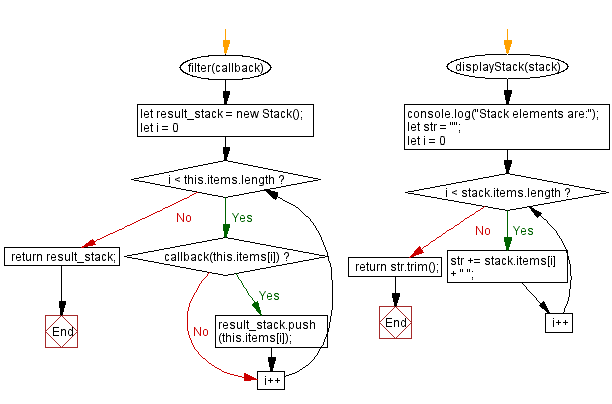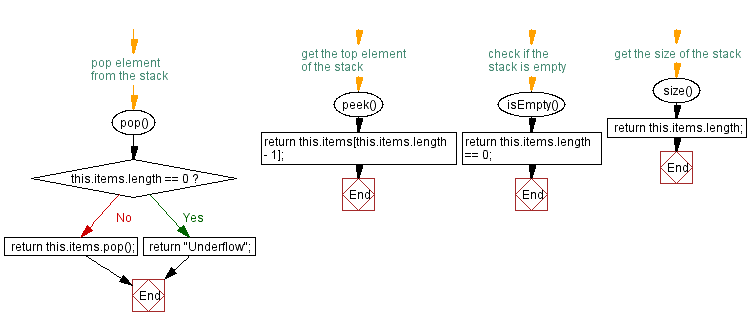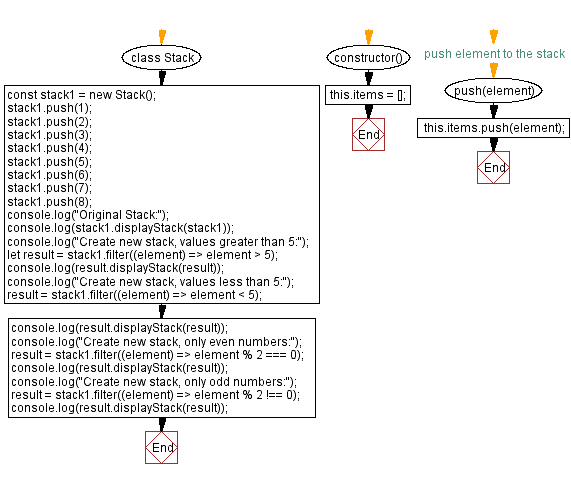# JavaScript Exercises: Remove elements from a stack that do not meet a condition

## JavaScript Stack: Exercise-35 with Solution

Write a JavaScript program that implements a stack and create a new stack by removing elements that do not satisfy a condition.

Sample Solution:

JavaScript Code:

``````class Stack {
constructor() {
this.items = [];
}
// push element to the stack
push(element) {
this.items.push(element);
}

// pop element from the stack
pop() {
if (this.items.length == 0) {
return "Underflow";
}
return this.items.pop();
}

// get the top element of the stack
peek() {
return this.items[this.items.length - 1];
}

// check if the stack is empty
isEmpty() {
return this.items.length == 0;
}

// get the size of the stack
size() {
return this.items.length;
}

filter(callback) {
let result_stack = new Stack();
for (let i = 0; i < this.items.length; i++) {
if (callback(this.items[i])) {
result_stack.push(this.items[i]);
}
}
return result_stack;
}

displayStack(stack) {
console.log("Stack elements are:");
let str = "";
for (let i = 0; i < stack.items.length; i++)
str += stack.items[i] + " ";
return str.trim();
}
}

const stack1 = new Stack();
stack1.push(1);
stack1.push(2);
stack1.push(3);
stack1.push(4);
stack1.push(5);
stack1.push(6);
stack1.push(7);
stack1.push(8);
console.log("Original Stack:");
console.log(stack1.displayStack(stack1));
console.log("Create new stack, values greater than 5:");
let result = stack1.filter((element) => element > 5);
console.log(result.displayStack(result));
console.log("Create new stack, values less than 5:");
result = stack1.filter((element) => element < 5);
console.log(result.displayStack(result));
console.log("Create new stack, only even numbers:");
result = stack1.filter((element) => element % 2 === 0);
console.log(result.displayStack(result));
console.log("Create new stack, only odd numbers:");
result = stack1.filter((element) => element % 2 !== 0);
console.log(result.displayStack(result));
```
```

Sample Output:

```Original Stack:
Stack elements are:
1 2 3 4 5 6 7 8
Create new stack, values greater than 5:
Stack elements are:
6 7 8
Create new stack, values less than 5:
Stack elements are:
1 2 3 4
Create new stack, only even numbers:
Stack elements are:
2 4 6 8
Create new stack, only odd numbers:
Stack elements are:
1 3 5 7
```

Flowchart:Live Demo:

See the Pen javascript-stack-exercise-35 by w3resource (@w3resource) on CodePen.

Improve this sample solution and post your code through Disqus

Stack Previous: Verify at least one element satisfy a condition.

What is the difficulty level of this exercise?

Test your Programming skills with w3resource's quiz.

﻿